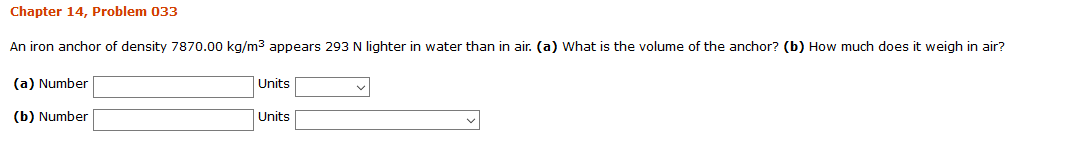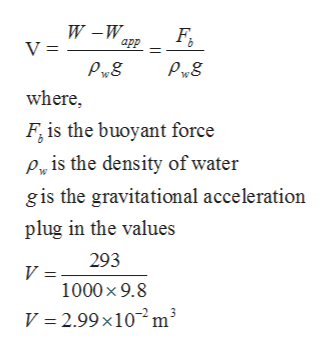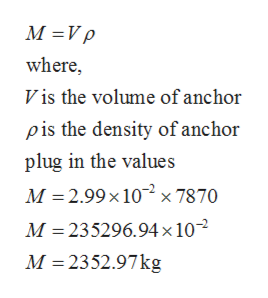# Chapter 14, Problem 033An iron anchor of density 7870.00 kg/m3 appears 293 N lighter in water than in air. (a) What is the volume of the anchor? (b) How much does it weigh in air?(a) NumberUnits(b) NumberUnits

Question
40 viewshelp_outlineImage TranscriptioncloseChapter 14, Problem 033 An iron anchor of density 7870.00 kg/m3 appears 293 N lighter in water than in air. (a) What is the volume of the anchor? (b) How much does it weigh in air? (a) Number Units (b) Number Units fullscreen
check_circle

Step 1

Given:

Density of the iron anchor = 7870 kg/m3

Buoyant force = 293 N

Density of water = 1000 kg/m3

Step 2

Part (a): Volume of anchor can be calculated as:help_outlineImage TranscriptioncloseW -W V = аp where F, is the buoyant force Pa is the density of water g is the gravitational acceleration plug in the values 293 V = 1000 x 9.8 V 2.99x10 m3 fullscreen
Step 3

Mass of anchor can be...help_outlineImage TranscriptioncloseM Vp where, Vis the volume of anchor pis the density of anchor plug in the values M 2.99x 10x 7870 M 235296.94x 10 M 2352.97kg fullscreen

### Want to see the full answer?

See Solution

#### Want to see this answer and more?

Solutions are written by subject experts who are available 24/7. Questions are typically answered within 1 hour.*

See Solution
*Response times may vary by subject and question.
Tagged in

### Mechanical Properties of Matter